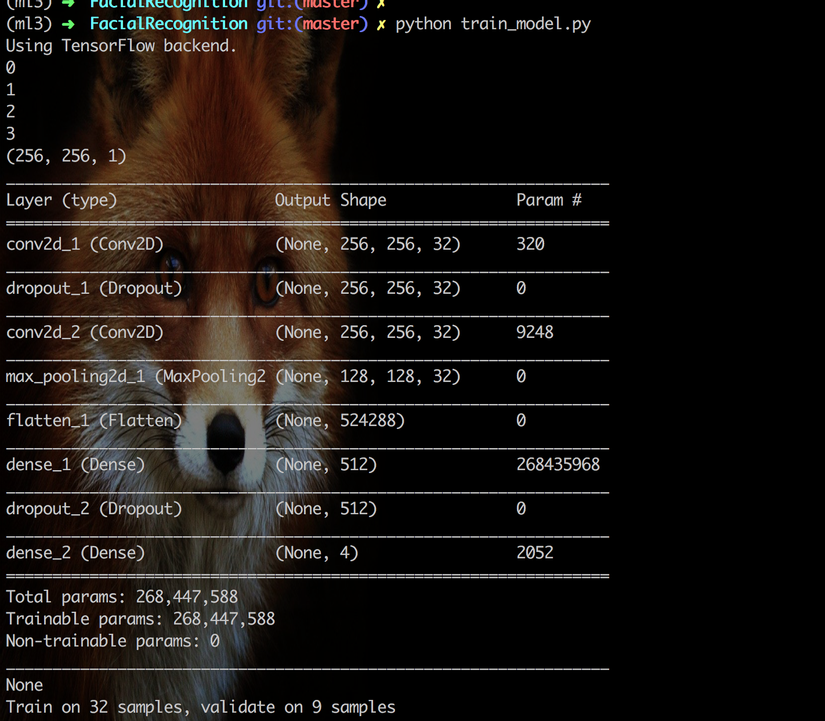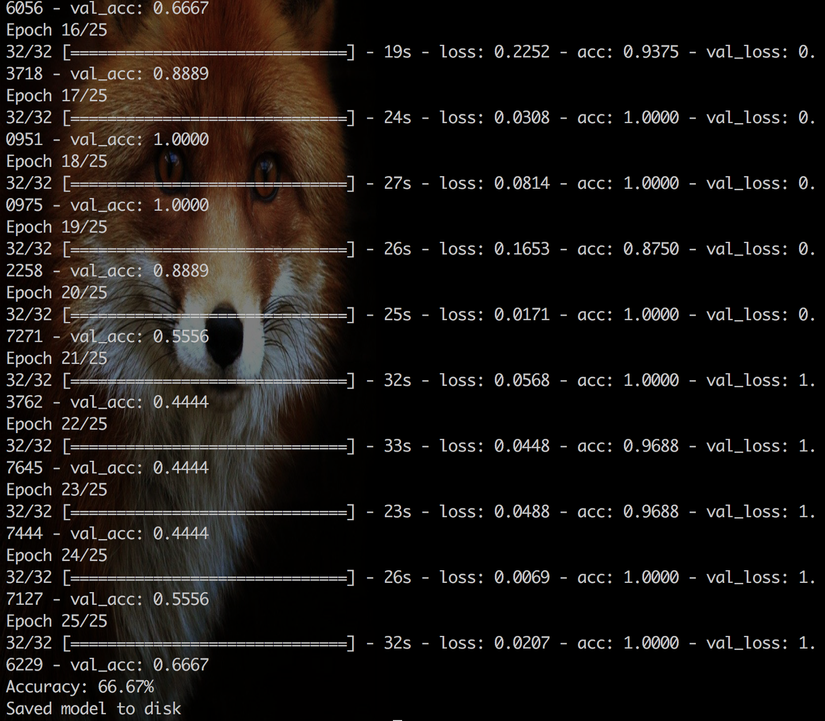+4

# Facial Recognition System: Face Recognition

We're about to complete our journey of building Facial Recognition System series. We're going to use a deep learning framework call Keras to create the learning model. Keras is a Python library for deep learning that wraps the powerful numerical libraries Theano and TensorFlow. However, we're not going explain much about this tool in this post and we'll create other post that talk about Keras later.

In this post, we will go over the following steps:

• How to load raw images data and convert it to format where we can fit to our model.
• How to create a simple Convolutional Neural Network
• Use the train model to recognize our face via webcame.

Let's rock.

### Prepare training/testing data

In my last post, we've already collect face images and store in folder `images`.

``````- images
- person1
- 0.png
- 1.png
- person2
- 0.png
- .......
``````

However, we cannot fit those files into our model directly. Because they accept only integer number or array of number only so we need to convert our image to into numpy array format. Here, how we convert raw images into numpy array list. First we import some package for read images.

``````import cv2
import os
import numpy as np

from keras.utils import np_utils
from sklearn.utils import shuffle
from sklearn.model_selection import train_test_split
``````

Next, we loop in folder `images` to get all image, then use `cv2.imread` to read and convert to gray color. Then, we store those images and label of people in array list.

``````# load and prepare data
people = ['Cristiano_Ronaldo', 'Jackie_Chan', 'Lionel_Messi', 'Rathanak']
num_classes = 4
img_data_list = []
labels = []
valid_images = [".jpg",".gif",".png"]

for index, person in enumerate(people):
print(index)
dir_path = 'images/' + person
for img_path in os.listdir(dir_path):
name, ext = os.path.splitext(img_path)
if ext.lower() not in valid_images:
continue

img_data = cv2.imread(dir_path + '/' + img_path)
# convert image to gray
img_data=cv2.cvtColor(img_data, cv2.COLOR_BGR2GRAY)
img_data_list.append(img_data)
labels.append(index)
``````

Next, we convert python array to numpy array and divde with `255.0` to improve training performance.

``````img_data = np.array(img_data_list)
img_data = img_data.astype('float32')

labels = np.array(labels ,dtype='int64')
# scale down(so easy to work with)
img_data /= 255.0
img_data= np.expand_dims(img_data, axis=4)
``````

Finaly, we shuffle our dataset for improving training accuracy.

``````# convert class labels to on-hot encoding
Y = np_utils.to_categorical(labels, num_classes)
# print(Y)

#Shuffle the dataset
x,y = shuffle(img_data,Y, random_state=2)

# Split the dataset
X_train, X_test, y_train, y_test = train_test_split(x, y, test_size=0.2, random_state=2)
``````

### Create a sample Convolutional Neural Network(CNN)

First, we need to import some packages:

``````from keras.utils import np_utils
from keras.models import Sequential
from keras.layers import Dense
from keras.layers import Dropout
from keras.layers import Flatten
from keras.constraints import maxnorm
from keras.optimizers import SGD
from keras.layers.convolutional import Conv2D
from keras.layers.convolutional import MaxPooling2D

# Saving and loading model and weights
from keras.models import model_from_json
from keras.models import load_model
``````

Then, we can init and compile our CNN model via Keras.

``````# Defining the model
input_shape=img_data.shape
print(input_shape)

model = Sequential()
model = Sequential()
model.add(Conv2D(32, (3, 3), input_shape=input_shape, padding='same', activation='relu', kernel_constraint=maxnorm(3)))

# Compile model
epochs = 25
lrate = 0.01
decay = lrate/epochs
sgd = SGD(lr=lrate, momentum=0.9, decay=decay, nesterov=False)
model.compile(loss='categorical_crossentropy', optimizer=sgd, metrics=['accuracy'])
print(model.summary())
``````

Lastly, we train model with our dataset and then save it into disk for later use.

``````# Fit the model
model.fit(X_train, y_train, validation_data=(X_test, y_test), epochs=epochs, batch_size=32)

# Final evaluation of the model
scores = model.evaluate(X_test, y_test, verbose=0)
print("Accuracy: %.2f%%" % (scores*100))

# serialize model to JSON
model_json = model.to_json()
with open("model.json", "w") as json_file:
json_file.write(model_json)
# serialize weights to HDF5
model.save_weights("model.h5")
print("Saved model to disk")
``````

We can combind those block into `train_model.py`:

``````import cv2
import os
import numpy as np

from keras.utils import np_utils
from keras.models import Sequential
from keras.layers import Dense
from keras.layers import Dropout
from keras.layers import Flatten
from keras.constraints import maxnorm
from keras.optimizers import SGD
from keras.layers.convolutional import Conv2D
from keras.layers.convolutional import MaxPooling2D

# Saving and loading model and weights
from keras.models import model_from_json
from keras.models import load_model

from sklearn.utils import shuffle
from sklearn.model_selection import train_test_split

# load and prepare data
people = ['Cristiano_Ronaldo', 'Jackie_Chan', 'Lionel_Messi', 'Rathanak']
num_classes = 4
img_data_list = []
labels = []
valid_images = [".jpg",".gif",".png"]

for index, person in enumerate(people):
print(index)
dir_path = 'images/' + person
for img_path in os.listdir(dir_path):
name, ext = os.path.splitext(img_path)
if ext.lower() not in valid_images:
continue

img_data = cv2.imread(dir_path + '/' + img_path)
# convert image to gray
img_data=cv2.cvtColor(img_data, cv2.COLOR_BGR2GRAY)
img_data_list.append(img_data)
labels.append(index)

img_data = np.array(img_data_list)
img_data = img_data.astype('float32')

labels = np.array(labels ,dtype='int64')
# scale down(so easy to work with)
img_data /= 255.0
img_data= np.expand_dims(img_data, axis=4)

# convert class labels to on-hot encoding
Y = np_utils.to_categorical(labels, num_classes)
# print(Y)

#Shuffle the dataset
x,y = shuffle(img_data,Y, random_state=2)

# Split the dataset
X_train, X_test, y_train, y_test = train_test_split(x, y, test_size=0.2, random_state=2)

# Defining the model
input_shape=img_data.shape
print(input_shape)

model = Sequential()
model = Sequential()
model.add(Conv2D(32, (3, 3), input_shape=input_shape, padding='same', activation='relu', kernel_constraint=maxnorm(3)))

# Compile model
epochs = 25
lrate = 0.01
decay = lrate/epochs
sgd = SGD(lr=lrate, momentum=0.9, decay=decay, nesterov=False)
model.compile(loss='categorical_crossentropy', optimizer=sgd, metrics=['accuracy'])
print(model.summary())

# Fit the model
model.fit(X_train, y_train, validation_data=(X_test, y_test), epochs=epochs, batch_size=32)

# Final evaluation of the model
scores = model.evaluate(X_test, y_test, verbose=0)
print("Accuracy: %.2f%%" % (scores*100))

# serialize model to JSON
model_json = model.to_json()
with open("model.json", "w") as json_file:
json_file.write(model_json)
# serialize weights to HDF5
model.save_weights("model.h5")
print("Saved model to disk")
``````

Let's train our model:And we got 66.67% of accuracy. Not bad.

### Test the train model

After we finish train and save our CNN model, we can load the model from disk and use it to recognize faces. First, we load CNN model from disk:

``````# Saving and loading model and weights
from keras.models import model_from_json
from keras.models import load_model

# load json and create model
json_file = open('model.json', 'r')
json_file.close()
# load weights into new model
print("Loaded model from disk")
``````

Next, we init face detection and load video from webcam.

``````print("[INFO] loading facial landmark predictor...")
detector = dlib.get_frontal_face_detector()
predictor = dlib.shape_predictor("model/shape_predictor_68_face_landmarks.dat")
fa = FaceAligner(predictor, desiredFaceWidth=256)

print("[INFO] camera sensor warming up...")
vs = VideoStream().start()
time.sleep(2.0)
``````

Then, we loop on every frame and convert to gray image. Then we detect faces in image frame.

``````    frame = vs.read()
frame = imutils.resize(frame, width=800)
height, width = frame.shape[:2]
gray_frame = cv2.cvtColor(frame, cv2.COLOR_BGR2GRAY)
# detect faces in the grayayscale frame
rects = detector(gray_frame, 0)
``````

Finally, we loop in every faces that we detected. Then, convert it into format where our model can predict.

``````faceAligned = fa.align(frame, gray_frame, rect)
faceAligned = cv2.cvtColor(faceAligned, cv2.COLOR_BGR2GRAY)
faceAligned = np.array(faceAligned)
faceAligned = faceAligned.astype('float32')
faceAligned /= 255.0
faceAligned= np.expand_dims([faceAligned], axis=4)

for index, value in enumerate(Y_pred):
result = people[index] + ': ' + str(int(value * 100)) + '%'
cv2.putText(frame, result, (10, 15 * (index + 1)), cv2.FONT_HERSHEY_SIMPLEX, 0.5, (0,0,0), 1)

# draw rect around face
(x,y,w,h) = face_utils.rect_to_bb(rect)
cv2.rectangle(frame, (x, y), (x+w, y+h), (0,0,255), 1)
# draw person name
result = np.argmax(Y_pred, axis=1)
cv2.putText(frame, people[result], (x, y - 5), cv2.FONT_HERSHEY_SIMPLEX, 0.5, (0,255,0), 1)
``````

Here, the full `test_model.py` code:

``````# test_model.py
from imutils.video import VideoStream
from imutils import face_utils
from imutils.face_utils import FaceAligner
import imutils
import time
import cv2
import dlib
import os
import numpy as np

# Saving and loading model and weights
from keras.models import model_from_json
from keras.models import load_model

# load json and create model
json_file = open('model.json', 'r')
json_file.close()
# load weights into new model
print("Loaded model from disk")

detector = dlib.get_frontal_face_detector()
predictor = dlib.shape_predictor("model/shape_predictor_68_face_landmarks.dat")
fa = FaceAligner(predictor, desiredFaceWidth=256)

print("[INFO] camera sensor warming up...")
vs = VideoStream().start()
time.sleep(2.0)

people = ['Cristiano_Ronaldo', 'Jackie_Chan', 'Lionel_Messi', 'Rathanak']
# loop over the frames from the video stream
while True:
key = cv2.waitKey(1) & 0xFF
# if the `q` key was pressed, break from the loop
if key == ord("q"):
break

frame = imutils.resize(frame, width=800)
height, width = frame.shape[:2]
gray_frame = cv2.cvtColor(frame, cv2.COLOR_BGR2GRAY)
# detect faces in the grayayscale frame
rects = detector(gray_frame, 0)

# loopop over the face detections
for rect in rects:
faceAligned = fa.align(frame, gray_frame, rect)
faceAligned = cv2.cvtColor(faceAligned, cv2.COLOR_BGR2GRAY)
faceAligned = np.array(faceAligned)
faceAligned = faceAligned.astype('float32')
faceAligned /= 255.0
faceAligned= np.expand_dims([faceAligned], axis=4)

for index, value in enumerate(Y_pred):
result = people[index] + ': ' + str(int(value * 100)) + '%'
cv2.putText(frame, result, (10, 15 * (index + 1)), cv2.FONT_HERSHEY_SIMPLEX, 0.5, (0,0,0), 1)

# draw rect around face
(x,y,w,h) = face_utils.rect_to_bb(rect)
cv2.rectangle(frame, (x, y), (x+w, y+h), (0,0,255), 1)
# draw person name
result = np.argmax(Y_pred, axis=1)
cv2.putText(frame, people[result], (x, y - 5), cv2.FONT_HERSHEY_SIMPLEX, 0.5, (0,255,0), 1)

# show the frame
cv2.imshow("Frame", frame)

# do a bit of cleanup
cv2.destroyAllWindows()
vs.stop()
``````

It's time to run `test_model.py`. Let's check out result belove:

Awesome, it's work.

### Last word

In this post, we finish the Facial Recognition System tutorial series by appying a deep learning algorithm to teach the machine to recognize on faces. However, its accuracy is just 66.67%, now it up to you to improve the learning model.